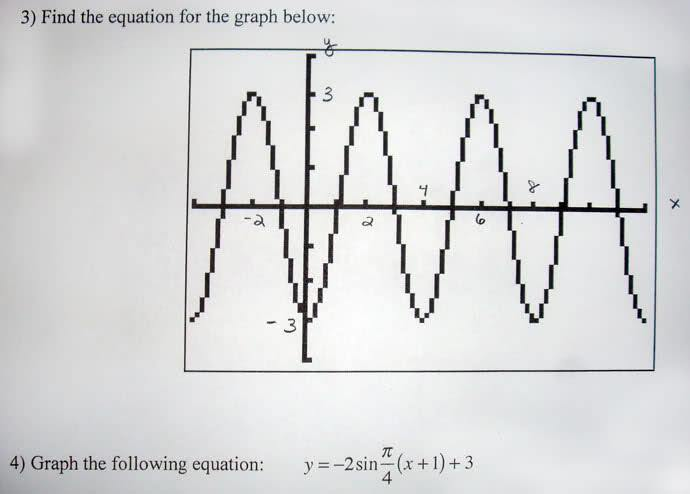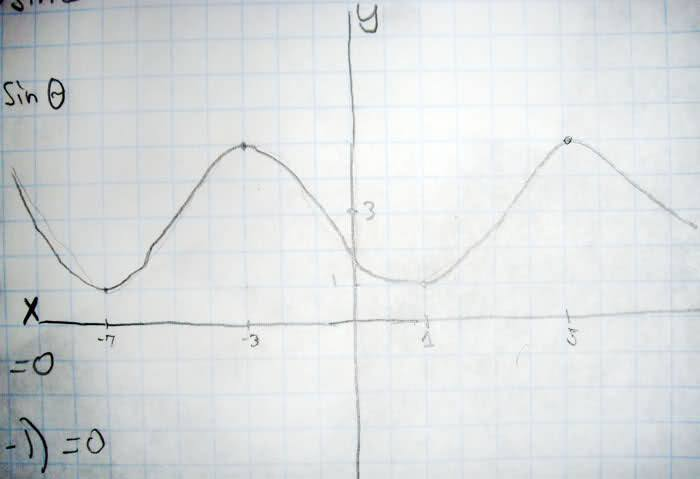# Two cos/sin wave graphing question

rought

## Homework Statement## The Attempt at a Solution

For #3 I need to finde the cos graph equation here's what I got: shift to the right 2, Amplitude = 3, b = π/2

equation: y=3cosπ/2(x-2)

I'm just not sure if i have it in the right format or if I'm even right

Attempt for #4 Amplitude=2 Shift left = 1 Shift up= 3n data[/b]

I'm just not sure if I am doing it right for both of them

For your first solution, you are correct, but if when you say "format" you are referring to a simpler form, you may wish to use the identity $$cos(x - \pi) = -cos(x)$$ to simplify the equation.